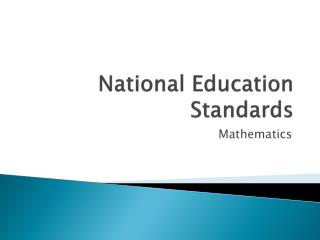DownloadDownload PresentationNational Education Standards

# National Education Standards

Download Presentation## National Education Standards

- - - - - - - - - - - - - - - - - - - - - - - - - - - E N D - - - - - - - - - - - - - - - - - - - - - - - - - - -
##### Presentation Transcript

1. National Education Standards Mathematics

2. Are standards hard to write? • Organization is difficult. • Categories are hard to pick. • How is it done?

3. Common Core has 10 content areas • Number • Quantity • Expressions • Equations • Functions • Modeling • Shape • Coordinates • Probability • Statistics

4. NAEP has 5 • Number properties and Operations • Measurement • Geometry • Data Analysis, Statistics, and Probability • Algebra

5. TIMSS has 3 for 4th grade 4 for 8th • 4th grade • Number • Geometric Shapes and Measures • Data Display • 8th grade • Number • Algebra • Geometry • Data and Chance

6. PISA has 4 areas • Space and shape • Change and relationships • Quantity • Uncertainty

7. So, organization is difficult. • But, it isn’t that important. • The structure of the standards doesn’t matter that much.

8. Anything easy about standards? • Picking content is easy! • Involve mathematicians. • Oops! What’s your image of mathematicians? • Your image is wrong! • Think: Mathematicians are 13th grade teachers. • Survey us. • Look at our placement tests. • Easy!

9. Content Choice Styles • PISA • They don’t bother. Not about math. • NAEP • Has content and kitchen sink. • Common Core • Thinks (minimal) college readiness. • TIMSS • Listens to mathematicians.

10. What else is easy? • Clarity is easy. • Use simple, precise, mathematical language.

11. TIMSS • Compare and order whole numbers. • Solve problems involving proportions. • Compute with fractions and decimals. • Solve problems involving percents and proportions. • Very nice, straightforward, clear.

12. Common Core, A step down. • Know when and how to use standard algorithms, and perform them flexibly, accurately, and efficiently. • Clean it up! • Know how to use standard algorithms efficiently. • Do you really have to mention “accurately?”

13. NAEP, much further down • Create and translate between different representations of algebraic expressions, equations, and inequalities (e.g., linear, quadratic, exponential, or trigonometric) using symbols, graphs, tables, diagrams, or written descriptions. • Yikes! Analyze by counting. • Forget create. Use only “translate.”

14. Three things • Expressions • Equations • inequalities

15. 4 functions • Linear • Quadratic • Exponential • trigonometric

16. 5 representations • Symbols • Graphs • Tables • Diagrams • Written descriptions

17. Translations among the 5 • 20 different translations • 4 different functions • 3 different mathematical relationships • Total: 240 standards in one sentence! • Oops, forgot “create” • 3x4x5=60 • Total: 300 standards in one sentence! • Clarity, simplicity? No!

18. PISA, lowest of all • Elegant computations • Recognizing shapes and patterns • Representing changes in a comprehensible form • Understanding the fundamental types of change • This is not guidance with clarity!

19. Biggest Difficulty – Setting Priorities • Some parts of standards are not as important as other parts of the same standard. • Some standards are not as important as other standards. • Some content areas are not as important as other content areas.

20. Recall NAEP • Create and translate between different representations of algebraic expressions, equations, and inequalities (e.g., linear, quadratic, exponential, or trigonometric) using symbols, graphs, tables, diagrams, or written descriptions. • Tables to written descriptions, not as important as symbols to graphs.

21. TIMSS standards comparison • Compute with fractions and decimals. • Use data from experiments to predict the chances of future outcomes. • One is essential math. • One is pretty important science.

22. Common Core areas • Probability and statistics are reasonable • But • They are 24% of the total standards. • That’s unreasonable.

23. Setting priorities is difficult to fix • TIMSS does it. • They tell you what percentage of their test will be on each area. • Others don’t succeed. • Even if you pick standards that are all absolutely essential, some take more time. • It is a difficult problem.